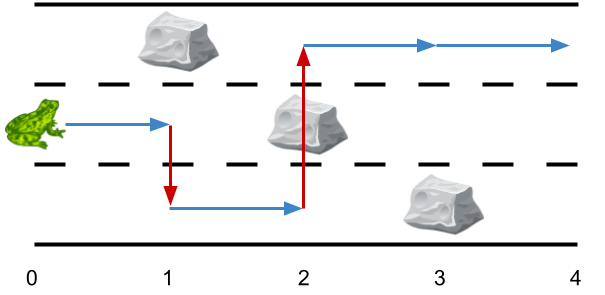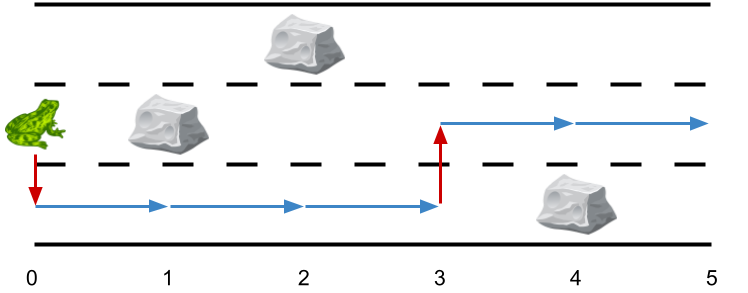Formatted question description: https://leetcode.ca/all/1824.html

# 1824. Minimum Sideway Jumps

Medium

## Description

There is a 3 lane road of length n that consists of n + 1 points labeled from 0 to n. A frog starts at point 0 in the second lane and wants to jump to point n. However, there could be obstacles along the way.

You are given an array obstacles of length n + 1 where each obstacles[i] (ranging from 0 to 3) describes an obstacle on the lane obstacles[i] at point i. If obstacles[i] == 0, there are no obstacles at point i. There will be at most one obstacle in the 3 lanes at each point.

• For example, if obstacles == 1, then there is an obstacle on lane 1 at point 2.

The frog can only travel from point i to point i + 1 on the same lane if there is not an obstacle on the lane at point i + 1. To avoid obstacles, the frog can also perform a side jump to jump to another lane (even if they are not adjacent) at the same point if there is no obstacle on the new lane.

• For example, the frog can jump from lane 3 at point 3 to lane 1 at point 3.

Return the minimum number of side jumps the frog needs to reach any lane at point n starting from lane 2 at point 0.

Note: There will be no obstacles on points 0 and n.

Example 1:Input: obstacles = [0,1,2,3,0]

Output: 2

Explanation: The optimal solution is shown by the arrows above. There are 2 side jumps (red arrows).

Note that the frog can jump over obstacles only when making side jumps (as shown at point 2).

Example 2:Input: obstacles = [0,1,1,3,3,0] Output: 0 Explanation: There are no obstacles on lane 2. No side jumps are required.

*Example 3:8Input: obstacles = [0,2,1,0,3,0]

Output: 2

Explanation: The optimal solution is shown by the arrows above. There are 2 side jumps.

Constraints:

• obstacles.length == n + 1
• 1 <= n <= 5 * 10^5
• 0 <= obstacles[i] <= 3
• obstacles == obstacles[n] == 0

## Solution

Use dynamic programming. Create a 2D array dp where dp[i][j] represents the minimum number of side jumps when reaching point i on lane j. Initially, dp = 0 and dp = dp = 1. For 1 <= i <= n and 1 <= j <= 3, dp[i][j] = INFINITY if there is an obstacle at point i on lane j. Otherwise, dp[i][j] is obtained among the minimum of dp[i - 1][j] and dp[i][k] where 1 <= k <= 3 and j != k. Finally, return the minimum of dp[n].

class Solution {
public int minSideJumps(int[] obstacles) {
int n = obstacles.length - 1;
final int INFINITY = n * 2;
int[][] dp = new int[n + 1];
dp = dp = 1;
for (int i = 1; i <= n; i++) {
int obstacle = obstacles[i];
for (int j = 1; j <= 3; j++)
dp[i][j] = dp[i - 1][j];
if (obstacle > 0)
dp[i][obstacle] = INFINITY;
for (int j = 1; j <= 3; j++) {
if (j != obstacle) {
for (int k = 1; k <= 3; k++) {
if (j != k && k != obstacle)
dp[i][j] = Math.min(dp[i][j], dp[i][k] + 1);
}
}
}
}
int minJumps = Integer.MAX_VALUE;
for (int i = 1; i <= 3; i++)
minJumps = Math.min(minJumps, dp[n][i]);
return minJumps;
}
}